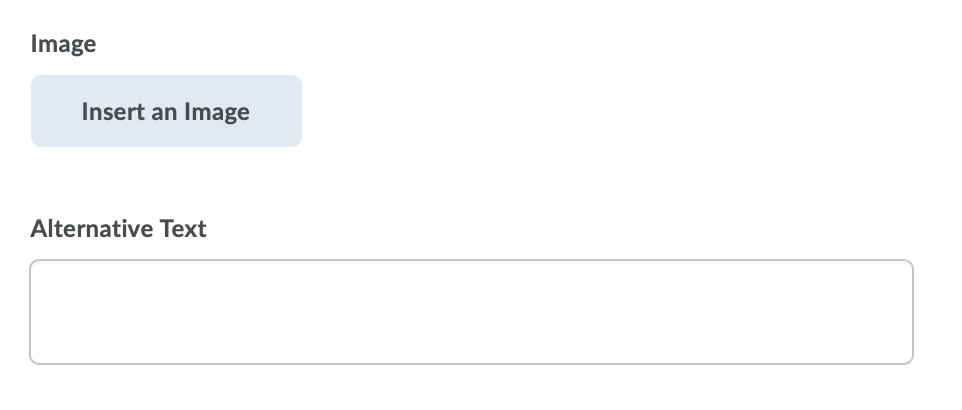# Create Multi-Short Answer Questions in the Question Library

Multi-short answer (MSA) questions require respondents to answer a multi-solution question and input their answers into individual input boxes. Respondent’s answers are checked against each possible answer stored in the answer fields. It is recommended that the required number of answers corresponds with the number of input boxes provided.

An MSA question’s maximum point value is reflected by a 100% weight. As a best practice, it is recommended that each possible answer’s weight calculation equals 100% divided by the number of answers required by the question.

Multi-short answer questions differ from short answer (SA) questions in that the multi-short answer question enables you to create multiple answer boxes that all relate to one answer set; short answer questions also support multiple answer boxes, but each requires a distinct set of possible answers. The short answer question type is ideal if you need to create a multi-part question that cannot share the same answer pool.

For example, the question “Name 3 state capitals” displays three input boxes to students. Each answer students submit is checked against 51 possible correct answers stored in the answer fields and each answer field has a weight of 33.3%.

Note: For a secure and consistent editing experience, JavaScript and non-standard HTML will automatically be filtered out. If your existing questions contain JavaScript or non-standard HTML, the questions continue to display as intended until an instructor edits and saves the quizzes, which removes the JavaScript and non-standard HTML.

1. On the nav bar, select the Activities menu, then click either Quizzes, Surveys, or Self Assessments. In the example below, we selected Quizzes.2. From the tool navigation, click Question Library.3. Select New.4. Select the question type:  Multi-Short Answer Question (MSA).
5. In the General area, enter your multi-short answer question details including:
6. Optional: Insert an Image and Image description (Alternative text)7. In the Options area, do the following:
• In the Input boxes field, enter the number of required answer fields you want your question to have. You can assign the number of rows and columns for each answer field.
• In the Answer fields, enter the correct answers for your question, the weight for each answer (you can set different weights if some solutions are more correct than others), and how you want the answers to be evaluated. There are three possible grading options:
• Text/Case Insensitive – Auto-grading searches for a matching character pattern in the answer text with or without letter case correctness.
• Case-Sensitive – Auto-grading searches for a matching character pattern in the answer text that must have letter case correctness. Proper capitalization is required.
• Regular Expression – Auto-grading uses meta-characters to search for one or more matching strings in the answer text’s character pattern. What you set as meta-character parameters helps determine letter case sensitivity. Learn more about Regular Expressions, see Understanding Regular Expressions.